# unit 2 hpc.pptx

15 de Mar de 2023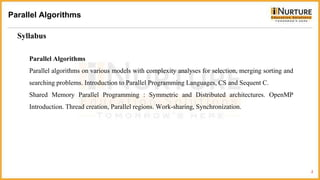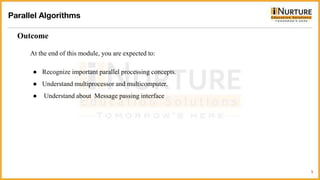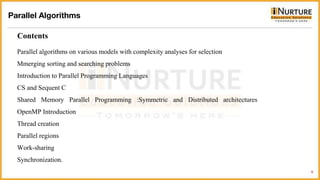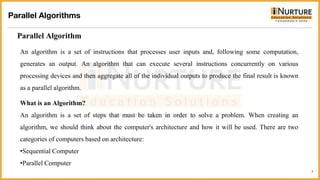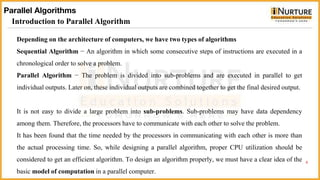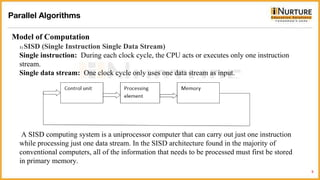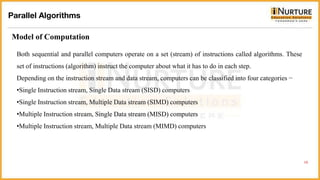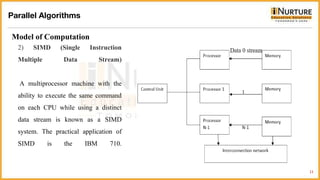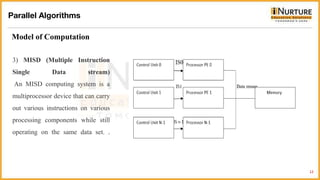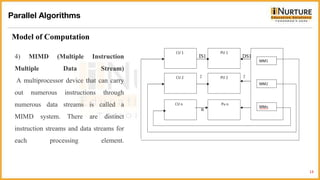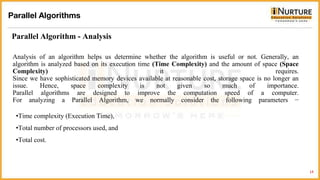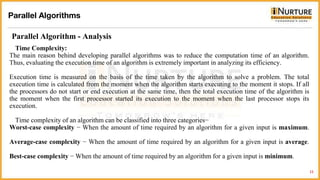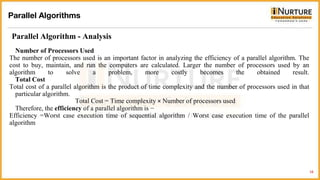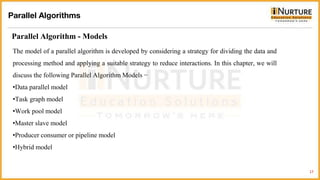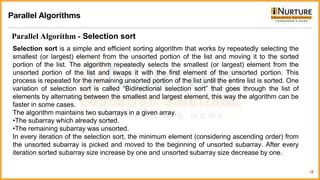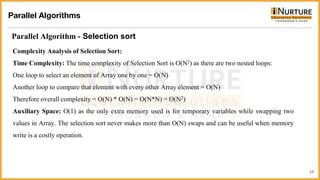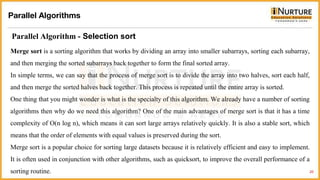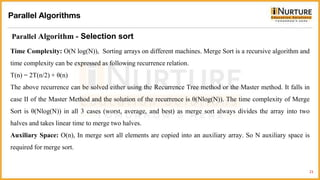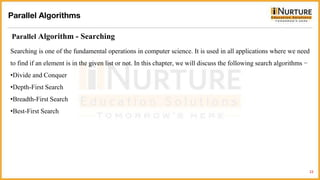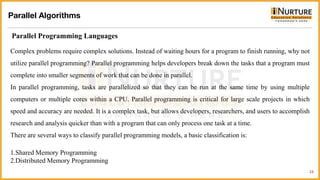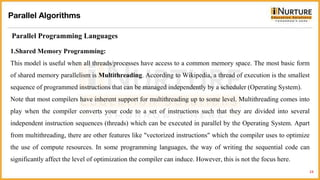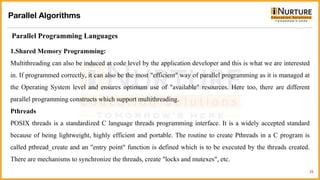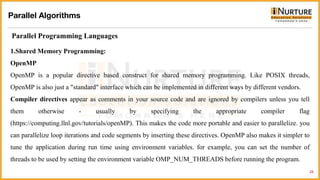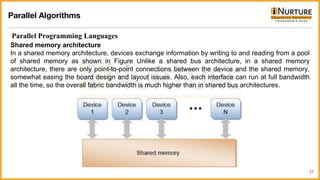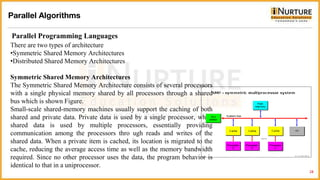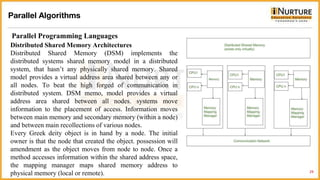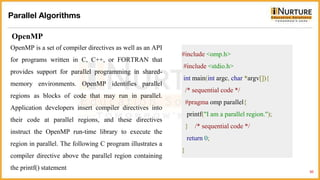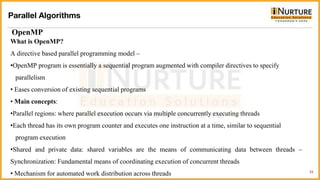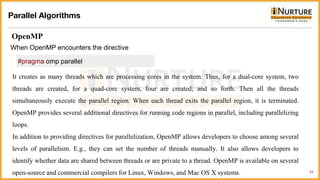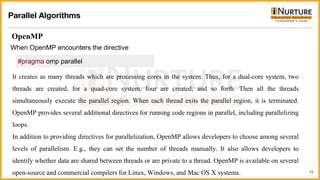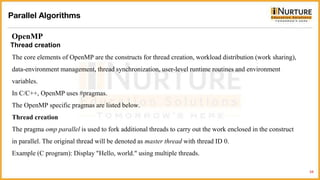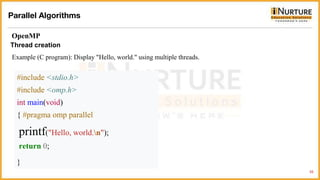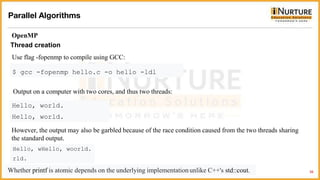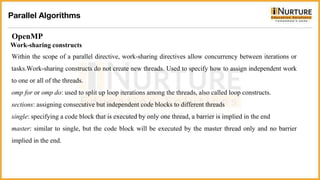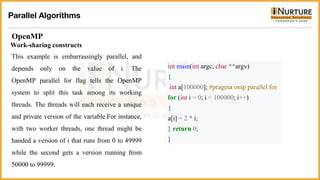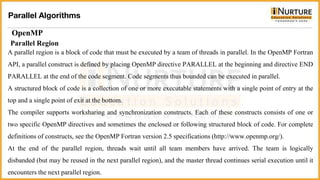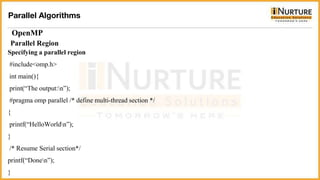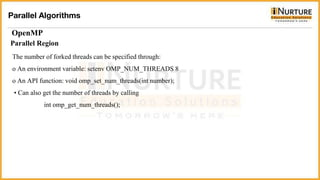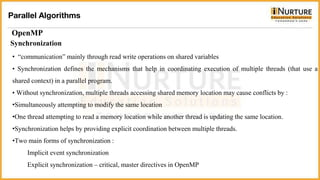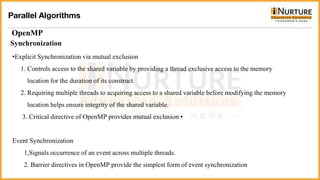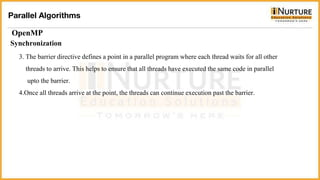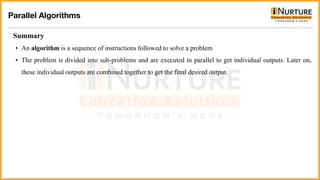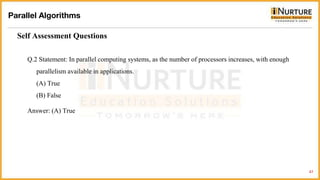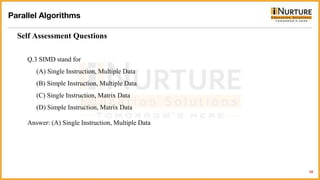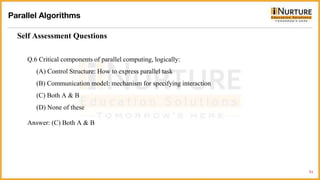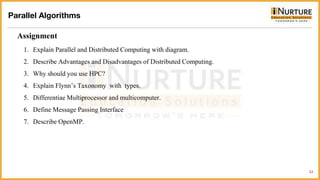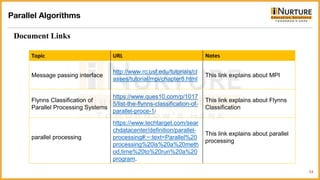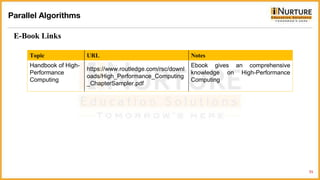1 de 55

### unit 2 hpc.pptx

• 1. Subject Name: High Performance Computing Module Number: 02 Module Name: Parallel Algorithms
• 2. 2 Syllabus Parallel Algorithms Parallel Algorithms Parallel algorithms on various models with complexity analyses for selection, merging sorting and searching problems. Introduction to Parallel Programming Languages, CS and Sequent C. Shared Memory Parallel Programming : Symmetric and Distributed architectures. OpenMP Introduction. Thread creation, Parallel regions. Work-sharing, Synchronization.
• 3. 3 Aim Parallel Algorithms It is a technique for processing enormous amounts of data quickly using a network of computers and storage devices. HPC makes it feasible to investigate and discover solutions to some of the biggest issues in business, engineering, and research.
• 4. 4 Objectives The Objectives of this module are • Identify different machine architecture • Learn about message passing interface Parallel Algorithms
• 5. 5 Outcome Parallel Algorithms At the end of this module, you are expected to: ● Recognize important parallel processing concepts. ● Understand multiprocessor and multicomputer. ● Understand about Message passing interface
• 6. 6 Contents Parallel Algorithms Parallel algorithms on various models with complexity analyses for selection Mmerging sorting and searching problems Introduction to Parallel Programming Languages CS and Sequent C Shared Memory Parallel Programming :Symmetric and Distributed architectures OpenMP Introduction Thread creation Parallel regions Work-sharing Synchronization.
• 7. 7 Parallel Algorithm Parallel Algorithms An algorithm is a set of instructions that processes user inputs and, following some computation, generates an output. An algorithm that can execute several instructions concurrently on various processing devices and then aggregate all of the individual outputs to produce the final result is known as a parallel algorithm. What is an Algorithm? An algorithm is a set of steps that must be taken in order to solve a problem. When creating an algorithm, we should think about the computer's architecture and how it will be used. There are two categories of computers based on architecture: •Sequential Computer •Parallel Computer
• 8. 8 Introduction to Parallel Algorithm Parallel Algorithms Depending on the architecture of computers, we have two types of algorithms Sequential Algorithm − An algorithm in which some consecutive steps of instructions are executed in a chronological order to solve a problem. Parallel Algorithm − The problem is divided into sub-problems and are executed in parallel to get individual outputs. Later on, these individual outputs are combined together to get the final desired output. It is not easy to divide a large problem into sub-problems. Sub-problems may have data dependency among them. Therefore, the processors have to communicate with each other to solve the problem. It has been found that the time needed by the processors in communicating with each other is more than the actual processing time. So, while designing a parallel algorithm, proper CPU utilization should be considered to get an efficient algorithm. To design an algorithm properly, we must have a clear idea of the basic model of computation in a parallel computer.
• 9. 1) SISD (Single Instruction Single Data Stream) Single instruction: During each clock cycle, the CPU acts or executes only one instruction stream. Single data stream: One clock cycle only uses one data stream as input. A SISD computing system is a uniprocessor computer that can carry out just one instruction while processing just one data stream. In the SISD architecture found in the majority of conventional computers, all of the information that needs to be processed must first be stored in primary memory. 9 Parallel Algorithms Model of Computation
• 10. 10 Model of Computation Both sequential and parallel computers operate on a set (stream) of instructions called algorithms. These set of instructions (algorithm) instruct the computer about what it has to do in each step. Depending on the instruction stream and data stream, computers can be classified into four categories − •Single Instruction stream, Single Data stream (SISD) computers •Single Instruction stream, Multiple Data stream (SIMD) computers •Multiple Instruction stream, Single Data stream (MISD) computers •Multiple Instruction stream, Multiple Data stream (MIMD) computers Parallel Algorithms
• 11. 2) SIMD (Single Instruction Multiple Data Stream) A multiprocessor machine with the ability to execute the same command on each CPU while using a distinct data stream is known as a SIMD system. The practical application of SIMD is the IBM 710. 11 Model of Computation Parallel Algorithms
• 12. 3) MISD (Multiple Instruction Single Data stream) An MISD computing system is a multiprocessor device that can carry out various instructions on various processing components while still operating on the same data set. . 12 Model of Computation Parallel Algorithms
• 13. 4) MIMD (Multiple Instruction Multiple Data Stream) A multiprocessor device that can carry out numerous instructions through numerous data streams is called a MIMD system. There are distinct instruction streams and data streams for each processing element. 13 Model of Computation Parallel Algorithms
• 14. Analysis of an algorithm helps us determine whether the algorithm is useful or not. Generally, an algorithm is analyzed based on its execution time (Time Complexity) and the amount of space (Space Complexity) it requires. Since we have sophisticated memory devices available at reasonable cost, storage space is no longer an issue. Hence, space complexity is not given so much of importance. Parallel algorithms are designed to improve the computation speed of a computer. For analyzing a Parallel Algorithm, we normally consider the following parameters − 14 Parallel Algorithm - Analysis Parallel Algorithms •Time complexity (Execution Time), •Total number of processors used, and •Total cost.
• 15. Time Complexity: The main reason behind developing parallel algorithms was to reduce the computation time of an algorithm. Thus, evaluating the execution time of an algorithm is extremely important in analyzing its efficiency. Execution time is measured on the basis of the time taken by the algorithm to solve a problem. The total execution time is calculated from the moment when the algorithm starts executing to the moment it stops. If all the processors do not start or end execution at the same time, then the total execution time of the algorithm is the moment when the first processor started its execution to the moment when the last processor stops its execution. Time complexity of an algorithm can be classified into three categories− Worst-case complexity − When the amount of time required by an algorithm for a given input is maximum. Average-case complexity − When the amount of time required by an algorithm for a given input is average. Best-case complexity − When the amount of time required by an algorithm for a given input is minimum. 15 Parallel Algorithm - Analysis Parallel Algorithms
• 16. Number of Processors Used The number of processors used is an important factor in analyzing the efficiency of a parallel algorithm. The cost to buy, maintain, and run the computers are calculated. Larger the number of processors used by an algorithm to solve a problem, more costly becomes the obtained result. Total Cost Total cost of a parallel algorithm is the product of time complexity and the number of processors used in that particular algorithm. Total Cost = Time complexity × Number of processors used Therefore, the efficiency of a parallel algorithm is − Efficiency =Worst case execution time of sequential algorithm / Worst case execution time of the parallel algorithm 16 Parallel Algorithm - Analysis Parallel Algorithms
• 17. 17 Parallel Algorithm - Models Parallel Algorithms The model of a parallel algorithm is developed by considering a strategy for dividing the data and processing method and applying a suitable strategy to reduce interactions. In this chapter, we will discuss the following Parallel Algorithm Models − •Data parallel model •Task graph model •Work pool model •Master slave model •Producer consumer or pipeline model •Hybrid model
• 18. 18 Parallel Algorithm - Selection sort Parallel Algorithms Selection sort is a simple and efficient sorting algorithm that works by repeatedly selecting the smallest (or largest) element from the unsorted portion of the list and moving it to the sorted portion of the list. The algorithm repeatedly selects the smallest (or largest) element from the unsorted portion of the list and swaps it with the first element of the unsorted portion. This process is repeated for the remaining unsorted portion of the list until the entire list is sorted. One variation of selection sort is called “Bidirectional selection sort” that goes through the list of elements by alternating between the smallest and largest element, this way the algorithm can be faster in some cases. The algorithm maintains two subarrays in a given array. •The subarray which already sorted. •The remaining subarray was unsorted. In every iteration of the selection sort, the minimum element (considering ascending order) from the unsorted subarray is picked and moved to the beginning of unsorted subarray. After every iteration sorted subarray size increase by one and unsorted subarray size decrease by one.
• 19. 19 Parallel Algorithm - Selection sort Parallel Algorithms Complexity Analysis of Selection Sort: Time Complexity: The time complexity of Selection Sort is O(N2) as there are two nested loops: One loop to select an element of Array one by one = O(N) Another loop to compare that element with every other Array element = O(N) Therefore overall complexity = O(N) * O(N) = O(N*N) = O(N2) Auxiliary Space: O(1) as the only extra memory used is for temporary variables while swapping two values in Array. The selection sort never makes more than O(N) swaps and can be useful when memory write is a costly operation.
• 20. 20 Parallel Algorithm - Selection sort Parallel Algorithms Merge sort is a sorting algorithm that works by dividing an array into smaller subarrays, sorting each subarray, and then merging the sorted subarrays back together to form the final sorted array. In simple terms, we can say that the process of merge sort is to divide the array into two halves, sort each half, and then merge the sorted halves back together. This process is repeated until the entire array is sorted. One thing that you might wonder is what is the specialty of this algorithm. We already have a number of sorting algorithms then why do we need this algorithm? One of the main advantages of merge sort is that it has a time complexity of O(n log n), which means it can sort large arrays relatively quickly. It is also a stable sort, which means that the order of elements with equal values is preserved during the sort. Merge sort is a popular choice for sorting large datasets because it is relatively efficient and easy to implement. It is often used in conjunction with other algorithms, such as quicksort, to improve the overall performance of a sorting routine.
• 21. 21 Parallel Algorithm - Selection sort Parallel Algorithms Time Complexity: O(N log(N)), Sorting arrays on different machines. Merge Sort is a recursive algorithm and time complexity can be expressed as following recurrence relation. T(n) = 2T(n/2) + θ(n) The above recurrence can be solved either using the Recurrence Tree method or the Master method. It falls in case II of the Master Method and the solution of the recurrence is θ(Nlog(N)). The time complexity of Merge Sort is θ(Nlog(N)) in all 3 cases (worst, average, and best) as merge sort always divides the array into two halves and takes linear time to merge two halves. Auxiliary Space: O(n), In merge sort all elements are copied into an auxiliary array. So N auxiliary space is required for merge sort.
• 22. 22 Parallel Algorithm - Searching Parallel Algorithms Searching is one of the fundamental operations in computer science. It is used in all applications where we need to find if an element is in the given list or not. In this chapter, we will discuss the following search algorithms − •Divide and Conquer •Depth-First Search •Breadth-First Search •Best-First Search
• 23. 23 Parallel Programming Languages Parallel Algorithms Complex problems require complex solutions. Instead of waiting hours for a program to finish running, why not utilize parallel programming? Parallel programming helps developers break down the tasks that a program must complete into smaller segments of work that can be done in parallel. In parallel programming, tasks are parallelized so that they can be run at the same time by using multiple computers or multiple cores within a CPU. Parallel programming is critical for large scale projects in which speed and accuracy are needed. It is a complex task, but allows developers, researchers, and users to accomplish research and analysis quicker than with a program that can only process one task at a time. There are several ways to classify parallel programming models, a basic classification is: 1.Shared Memory Programming 2.Distributed Memory Programming
• 24. 24 Parallel Programming Languages Parallel Algorithms 1.Shared Memory Programming: This model is useful when all threads/processes have access to a common memory space. The most basic form of shared memory parallelism is Multithreading. According to Wikipedia, a thread of execution is the smallest sequence of programmed instructions that can be managed independently by a scheduler (Operating System). Note that most compilers have inherent support for multithreading up to some level. Multithreading comes into play when the compiler converts your code to a set of instructions such that they are divided into several independent instruction sequences (threads) which can be executed in parallel by the Operating System. Apart from multithreading, there are other features like "vectorized instructions" which the compiler uses to optimize the use of compute resources. In some programming languages, the way of writing the sequential code can significantly affect the level of optimization the compiler can induce. However, this is not the focus here.
• 25. 25 Parallel Programming Languages Parallel Algorithms 1.Shared Memory Programming: Multithreading can also be induced at code level by the application developer and this is what we are interested in. If programmed correctly, it can also be the most "efficient" way of parallel programming as it is managed at the Operating System level and ensures optimum use of "available" resources. Here too, there are different parallel programming constructs which support multithreading. Pthreads POSIX threads is a standardized C language threads programming interface. It is a widely accepted standard because of being lightweight, highly efficient and portable. The routine to create Pthreads in a C program is called pthread_create and an "entry point" function is defined which is to be executed by the threads created. There are mechanisms to synchronize the threads, create "locks and mutexes", etc.
• 26. 26 Parallel Programming Languages Parallel Algorithms 1.Shared Memory Programming: OpenMP OpenMP is a popular directive based construct for shared memory programming. Like POSIX threads, OpenMP is also just a "standard" interface which can be implemented in different ways by different vendors. Compiler directives appear as comments in your source code and are ignored by compilers unless you tell them otherwise - usually by specifying the appropriate compiler flag (https://computing.llnl.gov/tutorials/openMP). This makes the code more portable and easier to parallelize. you can parallelize loop iterations and code segments by inserting these directives. OpenMP also makes it simpler to tune the application during run time using environment variables. for example, you can set the number of threads to be used by setting the environment variable OMP_NUM_THREADS before running the program.
• 27. 27 Parallel Programming Languages Parallel Algorithms Shared memory architecture In a shared memory architecture, devices exchange information by writing to and reading from a pool of shared memory as shown in Figure Unlike a shared bus architecture, in a shared memory architecture, there are only point-to-point connections between the device and the shared memory, somewhat easing the board design and layout issues. Also, each interface can run at full bandwidth all the time, so the overall fabric bandwidth is much higher than in shared bus architectures.
• 28. 28 Parallel Programming Languages Parallel Algorithms There are two types of architecture •Symmetric Shared Memory Architectures •Distributed Shared Memory Architectures Symmetric Shared Memory Architectures The Symmetric Shared Memory Architecture consists of several processors with a single physical memory shared by all processors through a shared bus which is shown Figure. Small-scale shared-memory machines usually support the caching of both shared and private data. Private data is used by a single processor, while shared data is used by multiple processors, essentially providing communication among the processors thro ugh reads and writes of the shared data. When a private item is cached, its location is migrated to the cache, reducing the average access time as well as the memory bandwidth required. Since no other processor uses the data, the program behavior is identical to that in a uniprocessor.
• 29. 29 Parallel Programming Languages Parallel Algorithms Distributed Shared Memory Architectures Distributed Shared Memory (DSM) implements the distributed systems shared memory model in a distributed system, that hasn’t any physically shared memory. Shared model provides a virtual address area shared between any or all nodes. To beat the high forged of communication in distributed system. DSM memo, model provides a virtual address area shared between all nodes. systems move information to the placement of access. Information moves between main memory and secondary memory (within a node) and between main recollections of various nodes. Every Greek deity object is in hand by a node. The initial owner is that the node that created the object. possession will amendment as the object moves from node to node. Once a method accesses information within the shared address space, the mapping manager maps shared memory address to physical memory (local or remote).
• 30. 30 OpenMP Parallel Algorithms OpenMP is a set of compiler directives as well as an API for programs written in C, C++, or FORTRAN that provides support for parallel programming in shared- memory environments. OpenMP identifies parallel regions as blocks of code that may run in parallel. Application developers insert compiler directives into their code at parallel regions, and these directives instruct the OpenMP run-time library to execute the region in parallel. The following C program illustrates a compiler directive above the parallel region containing the printf() statement #include <omp.h> #include <stdio.h> int main(int argc, char *argv[]){ /* sequential code */ #pragma omp parallel{ printf("I am a parallel region."); } /* sequential code */ return 0; }
• 31. 31 OpenMP Parallel Algorithms What is OpenMP? A directive based parallel programming model – •OpenMP program is essentially a sequential program augmented with compiler directives to specify parallelism • Eases conversion of existing sequential programs • Main concepts: •Parallel regions: where parallel execution occurs via multiple concurrently executing threads •Each thread has its own program counter and executes one instruction at a time, similar to sequential program execution •Shared and private data: shared variables are the means of communicating data between threads – Synchronization: Fundamental means of coordinating execution of concurrent threads • Mechanism for automated work distribution across threads
• 32. 32 OpenMP Parallel Algorithms When OpenMP encounters the directive #pragma omp parallel It creates as many threads which are processing cores in the system. Thus, for a dual-core system, two threads are created, for a quad-core system, four are created; and so forth. Then all the threads simultaneously execute the parallel region. When each thread exits the parallel region, it is terminated. OpenMP provides several additional directives for running code regions in parallel, including parallelizing loops. In addition to providing directives for parallelization, OpenMP allows developers to choose among several levels of parallelism. E.g., they can set the number of threads manually. It also allows developers to identify whether data are shared between threads or are private to a thread. OpenMP is available on several open-source and commercial compilers for Linux, Windows, and Mac OS X systems.
• 33. 33 OpenMP Parallel Algorithms When OpenMP encounters the directive #pragma omp parallel It creates as many threads which are processing cores in the system. Thus, for a dual-core system, two threads are created, for a quad-core system, four are created; and so forth. Then all the threads simultaneously execute the parallel region. When each thread exits the parallel region, it is terminated. OpenMP provides several additional directives for running code regions in parallel, including parallelizing loops. In addition to providing directives for parallelization, OpenMP allows developers to choose among several levels of parallelism. E.g., they can set the number of threads manually. It also allows developers to identify whether data are shared between threads or are private to a thread. OpenMP is available on several open-source and commercial compilers for Linux, Windows, and Mac OS X systems.
• 35. 35 OpenMP Parallel Algorithms Thread creation Example (C program): Display "Hello, world." using multiple threads. #include <stdio.h> #include <omp.h> int main(void) { #pragma omp parallel printf("Hello, world.n"); return 0; }
• 36. 36 OpenMP Parallel Algorithms Thread creation Use flag -fopenmp to compile using GCC: \$ gcc -fopenmp hello.c -o hello -ldl Output on a computer with two cores, and thus two threads: Hello, world. Hello, world. However, the output may also be garbled because of the race condition caused from the two threads sharing the standard output. Hello, wHello, woorld. rld. Whether printf is atomic depends on the underlying implementationunlike C++'s std::cout.
• 37. OpenMP Parallel Algorithms Work-sharing constructs Within the scope of a parallel directive, work-sharing directives allow concurrency between iterations or tasks.Work-sharing constructs do not create new threads. Used to specify how to assign independent work to one or all of the threads. omp for or omp do: used to split up loop iterations among the threads, also called loop constructs. sections: assigning consecutive but independent code blocks to different threads single: specifying a code block that is executed by only one thread, a barrier is implied in the end master: similar to single, but the code block will be executed by the master thread only and no barrier implied in the end.
• 38. OpenMP Parallel Algorithms Work-sharing constructs This example is embarrassingly parallel, and depends only on the value of i. The OpenMP parallel for flag tells the OpenMP system to split this task among its working threads. The threads will each receive a unique and private version of the variable.For instance, with two worker threads, one thread might be handed a version of i that runs from 0 to 49999 while the second gets a version running from 50000 to 99999. int main(int argc, char **argv) { int a; #pragma omp parallel for for (int i = 0; i < 100000; i++) { a[i] = 2 * i; } return 0; }
• 39. OpenMP Parallel Algorithms Parallel Region A parallel region is a block of code that must be executed by a team of threads in parallel. In the OpenMP Fortran API, a parallel construct is defined by placing OpenMP directive PARALLEL at the beginning and directive END PARALLEL at the end of the code segment. Code segments thus bounded can be executed in parallel. A structured block of code is a collection of one or more executable statements with a single point of entry at the top and a single point of exit at the bottom. The compiler supports worksharing and synchronization constructs. Each of these constructs consists of one or two specific OpenMP directives and sometimes the enclosed or following structured block of code. For complete definitions of constructs, see the OpenMP Fortran version 2.5 specifications (http://www.openmp.org/). At the end of the parallel region, threads wait until all team members have arrived. The team is logically disbanded (but may be reused in the next parallel region), and the master thread continues serial execution until it encounters the next parallel region.
• 40. OpenMP Parallel Algorithms Parallel Region Specifying a parallel region #include<omp.h> int main(){ print(“The output:n”); #pragma omp parallel /* define multi-thread section */ { printf(“HelloWorldn”); } /* Resume Serial section*/ printf(“Donen”); }
• 41. OpenMP Parallel Algorithms Parallel Region The number of forked threads can be specified through: o An environment variable: setenv OMP_NUM_THREADS 8 o An API function: void omp_set_num_threads(int number); • Can also get the number of threads by calling int omp_get_num_threads();
• 42. OpenMP Parallel Algorithms Synchronization • “communication” mainly through read write operations on shared variables • Synchronization defines the mechanisms that help in coordinating execution of multiple threads (that use a shared context) in a parallel program. • Without synchronization, multiple threads accessing shared memory location may cause conflicts by : •Simultaneously attempting to modify the same location •One thread attempting to read a memory location while another thread is updating the same location. •Synchronization helps by providing explicit coordination between multiple threads. •Two main forms of synchronization : Implicit event synchronization Explicit synchronization – critical, master directives in OpenMP
• 43. OpenMP Parallel Algorithms Synchronization •Explicit Synchronization via mutual exclusion 1. Controls access to the shared variable by providing a thread exclusive access to the memory location for the duration of its construct. 2. Requiring multiple threads to acquiring access to a shared variable before modifying the memory location helps ensure integrity of the shared variable. 3. Critical directive of OpenMP provides mutual exclusion • Event Synchronization 1,Signals occurrence of an event across multiple threads. 2. Barrier directives in OpenMP provide the simplest form of event synchronization
• 44. OpenMP Parallel Algorithms Synchronization 3. The barrier directive defines a point in a parallel program where each thread waits for all other threads to arrive. This helps to ensure that all threads have executed the same code in parallel upto the barrier. 4.Once all threads arrive at the point, the threads can continue execution past the barrier.
• 45. • An algorithm is a sequence of instructions followed to solve a problem • The problem is divided into sub-problems and are executed in parallel to get individual outputs. Later on, these individual outputs are combined together to get the final desired output. Summary Parallel Algorithms
• 46. 46 Self Assessment Questions Q.1 A parallel computing system consists of multiple processor that communicate with each other using a ___. (A) Allocated memory (B) Shared memory (C) Network (D) None of the above Answer: (B) Shared memory Parallel Algorithms
• 47. 47 Self Assessment Questions Q.2 Statement: In parallel computing systems, as the number of processors increases, with enough parallelism available in applications. (A) True (B) False Answer: (A) True Parallel Algorithms
• 48. 48 Self Assessment Questions Q.3 SIMD stand for (A) Single Instruction, Multiple Data (B) Simple Instruction, Multiple Data (C) Single Instruction, Matrix Data (D) Simple Instruction, Matrix Data Answer: (A) Single Instruction, Multiple Data Parallel Algorithms
• 49. 49 Self Assessment Questions Q.4 GPU stand for (A) Graphics Processing Unit (B) Graphical Processing Unit (C) Graphics Processed Unit (D) Graphical Processed Unit Answer: (A) Graphics Processing Unit Parallel Algorithms
• 50. 50 Self Assessment Questions Q.5 Interconnection network classify as (A) Static network (B) Dynamic network (C) Both A & B (D) None of these Answer: (C) Both A & B Parallel Algorithms
• 51. 51 Self Assessment Questions Q.6 Critical components of parallel computing, logically: (A) Control Structure: How to express parallel task (B) Communication model: mechanism for specifying interaction (C) Both A & B (D) None of these Answer: (C) Both A & B Parallel Algorithms
• 52. 52 Assignment 1. Explain Parallel and Distributed Computing with diagram. 2. Describe Advantages and Disadvantages of Distributed Computing. 3. Why should you use HPC? 4. Explain Flynn’s Taxonomy with types. 5. Differentiae Multiprocessor and multicomputer. 6. Define Message Passing Interface 7. Describe OpenMP. Parallel Algorithms
• 53. Topic URL Notes Message passing interface http://www.rc.usf.edu/tutorials/cl asses/tutorial/mpi/chapter8.html This link explains about MPI Flynns Classification of Parallel Processing Systems https://www.ques10.com/p/1017 5/list-the-flynns-classification-of- parallel-proce-1/ This link explains about Flynns Classification parallel processing https://www.techtarget.com/sear chdatacenter/definition/parallel- processing#:~:text=Parallel%20 processing%20is%20a%20meth od,time%20to%20run%20a%20 program. This link explains about parallel processing Document Links 53 Parallel Algorithms
• 54. Topic URL Notes Parallel Algorithms hhttps://archive.nptel.ac.in/courses/10 6/105/106105033/ Explains about pos tagging and stop word What is Parallel Algorithms https://www.youtube.com/watch?v=0 biElmaDfFs This video talks about Parallel Algorithms basic Video Links 54 Parallel Algorithms
• 55. Topic URL Notes Handbook of High- Performance Computing https://www.routledge.com/rsc/downl oads/High_Performance_Computing _ChapterSampler.pdf Ebook gives an comprehensive knowledge on High-Performance Computing E-Book Links 55 Parallel Algorithms